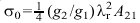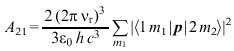# What is the relationship between Einstein's coefficients

## Einstein coefficient

Einstein coefficient, a parameter characteristic of a type of atom or molecule, which essentially determines the emission or absorption rate (number of photons emitted or absorbed in the unit of time) of a system of atoms or molecules.

1) spontaneous emission: The E. for spontaneous emission A.21 indicates the probability that an excited atom or molecule will spontaneously emit a photon in the unit of time, whereby it changes from a higher energy level 2 to a lower level 1. The emission takes place at the resonance frequency νr of the atomic system, i.e. in the case of the Planck quantum of action H divided level distance. The spontaneous emission rate is then the product of A.21 and the number of excited particles. The reciprocal of A.21 is, if no further transitions starting from the upper level are possible, equal to the mean service life of level 2.

2) induced emission: The E. for induced emission B.21 with which - at the resonance frequency νr taken - energy density related to the unit of the frequency interval ur) the already existing radiation (assumed to be isotropic) multiplied, the corresponding probability for induced emission of a photon.

3) absorption: The E. for absorption B.12 there, with ur) is multiplied, the probability that an atom located in a lower energy level 1 will move to a higher level 2 with the absorption of a photon in the unit of time.

From thermodynamic considerations, Einstein derived the following general relationships: B.21=A.21c3/ (8πHνr3), in which c the speed of light means as well B.12=(G2/G1) B.21 With G1 and G2 as the degree of degeneracy (number of sub-levels) of the levels. Note that the numerical values ​​for B.12 and B.21 on the choice of the reference quantities (in addition to the energy density, the energy flow density is also used, whereby the quantities mentioned can be related to the unit of the interval of frequency, angular frequency or wavelength) In the case of directed radiation, there is a connectionbetween A.21 and the total absorption cross section σ0 (Absorption coefficient), where λr the resonance wavelength λr=c/ νr designated. The E. A.21 is also related to the oscillator strength.

According to quantum theory, it results for A.21 the following expression